Question

Find the x- and y-intercepts of the graph of -x +y = 8. State your answers as
whole numbers or as improper fractions in simplest form.

1.Given:

The equation is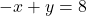To find:

The x- and y-intercepts.

Solution:

We have,Put x=0 in the given equation to find the y-intercept.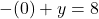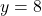Put y=0 in the given equation to find the x-intercept.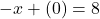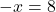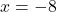Therefore, the x-intercept is -8 and the y-intercept is 8.